Uh Oh! It seems you’re using an Ad blocker!

Since we’ve struggled a lot to makes online calculations for you, we are appealing to you to grant us by disabling the Ad blocker for this domain.# Circumcenter Calculator

Enter x1

Enter y1

Enter x2

Enter y2

Enter x3

Enter y3

Table of Content

 1 What Is A Circumcenter? 2 Characteristics Of A Circumcenter: 3 How To Construct A Circumcenter? 4 How To Find Circumcenter? 5 Does every triangle has circumcenter? 6 What is meant by the circumference of a circle? 7 What is an orthocenter? 8 What do you mean by circumcircle?

Get the Widget!

Add this calculator to your site and lets users to perform easy calculations.

Feedback

How easy was it to use our calculator? Did you face any problem, tell us!

An online circumcenter calculator is specially designed to help you in finding the coordinates of a circumcenter of a triangle accurately. But, wait a second! Before you start using our free calculator, give a read to this organic content to better understand the concept.

Jump down!

## What Is A Circumcenter?

“A particular point where all the right bisectors of a triangle intersect each other is called the circumcenter of a triangle”.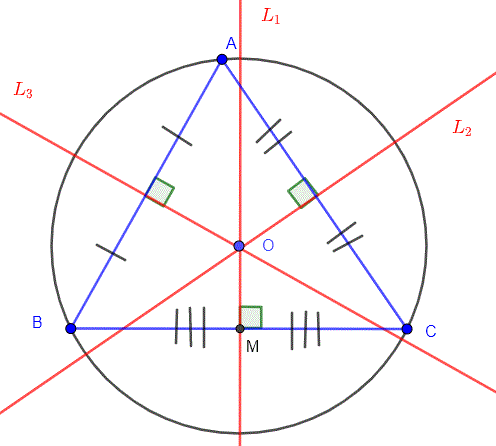What do you see in the above picture? Do not panic as we are going to explain each and everything for you.

In the above pictorial representation:

• A triangle has been drawn inside a circle.
• A, B and C are the three vertices of the triangle.
• L1, L2, and L3 are the right bisectors drawn at sides BC, AC, and AB, respectively.
• M represents the midpoint of the lines AB, BC and CA.
• Finally, we have a point O where all the bisectors are intersecting and is called the circumcenter.

Here, our free online circumcenter calculator find the circumcenter of the triangle absolutely for free.

### Characteristics Of A Circumcenter:

You must take into account some basic characteristics of the circumcenter that are as follows:

#### Characteristic # 01:

Circumcenter is a point from which all the three vertices of a triangle are exactly at the same distance.

⊿$$OA = OB = OC$$

#### Characteristic # 02:

When O and A are on the different sides of the triangle and the measurement of angle A (∠A) is obtuse, then we have:

$$∠BOC = 2( 180° – ∠A)$$

#### Characteristic # 03:

When we draw new triangles by joining their vertices to the circumcenter, they are actually isosceles triangles.

#### Characteristic # 04:

Whenever O and A are on the same side of BC and the measurement of angle A(∠A) is acute, then we have:

$$∠BOC = 2 ∠A$$

#### Characteristic # 05:

A circumcenter has different locations for various types of triangles as described below:

Acute Triangle:

The circumcenter of a right triangle lies inside of it.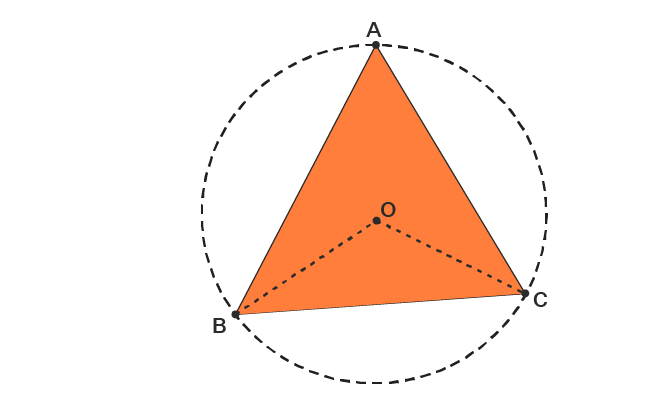Obtuse Triangle:

The circumcenter of an obtuse triangle lies outside the triangle.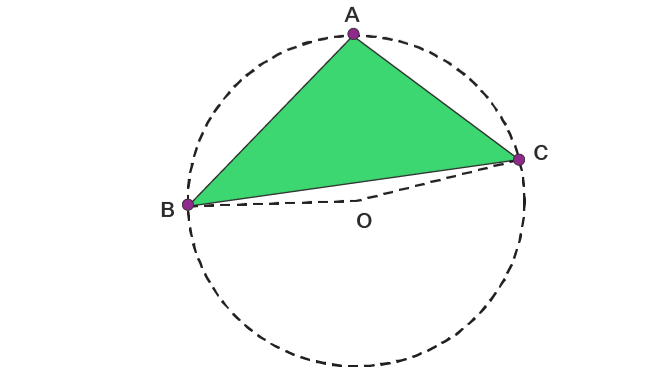Right Triangle:

The circumcenter of a right triangle lies on the hypotenuse of the triangle.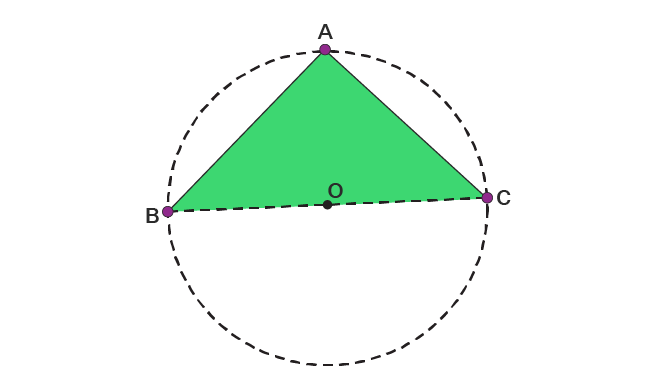### How To Construct A Circumcenter?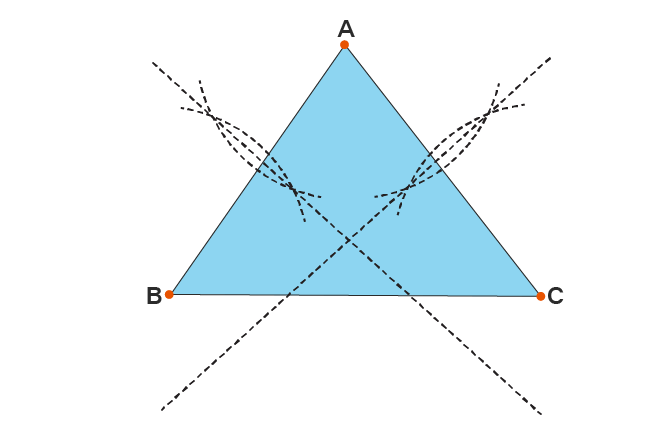Practically, it is very easy to construct a circumcenter. Keep an eye on the steps below to perform this task:

• First of all, you need a compass.
• After that, draw a triangle having equal sides.
• Now, take your compass and draw right bisectors on all the three sides of the triangle.
• After doing so, extend all bisectors until they intersect at a common point O. this point is called the circumcenter.

### How To Find Circumcenter?

You could now determine the coordinates of the circumcenter with the help of a free online circumcenter calculator. But when it comes to manual calculations, you need to adopt an accurate method to enumerate the results. What about solving an example to better get a grip!

Example:

How to find the circumcenter of a triangle with coordinates as follows:

$$A(5, 1), B(2, 1), C(6, 1)$$

Solution:

Now, you need to follow a series of steps to find the circumcenter of the triangle. These include:

Step # 01:

First step includes the determination of the midpoint for each side of the triangle.

Let us suppose that M is the midpoint of the side AB and N is the midpoint of the side BC, respectively. So, we have:

$$M = (\frac{x_{1} + x_{2}}{2} , \frac{y_{1} + y_{2}}{2})$$

$$M = (\frac{5 + 2}{2} , \frac{1 + 1}{2})$$

$$M = (\frac{7}{2} , \frac{2}{2})$$

$$M = \left(3.5, 1\right)$$

Now, we have:

$$N = (\frac{x_{1} + x_{2}}{2} , \frac{y_{1} + y_{2}}{2})$$

$$N= (\frac{6 + 2}{2} , \frac{1 + 1}{2})$$

$$N = (\frac{8}{2} , \frac{2}{2})$$

$$N = \left(4, 1\right)$$

Step # 02:

Now, you need to determine the slopes of each side and perpendicular to each side as below:

$$Slope of the side AB = [\frac{\left(y_{2} – y_{1}\right)}{\left(x_{2} – x_{1}\right)}]$$

$$Slope of the side AB = [\frac{\left(1 – 1\right)}{\left(2 – 5\right)}]$$

$$Slope of the side AB = \frac{0}{-3}$$

$$Slope of the side AB = 0$$

$$Slope of the perpendicular to AB = m = \frac{-1}{Slope of the side AB}$$

$$Slope of the perpendicular to AB = m = \frac{-1}{0}$$

$$Slope of the perpendicular to AB = m = 0$$

$$m = 0$$

Now, we have:

$$Slope of the side BC = [\frac{\left(y_{2} – y_{1}\right)}{\left(x_{2} – x_{1}\right)}]$$

$$Slope of the side BC = [\frac{\left(1 – 1\right)}{\left(6 – 2\right)}]$$

$$Slope of the side BC = [\frac{0}{4]$$

$$Slope of the side BC = 0$$

$$Slope of the perpendicular to BC = m = \frac{-1}{Slope of the side BC}$$

$$Slope of the perpendicular to BC = m = \frac{-1}{0}$$

$$Slope of the perpendicular to BC = m = 0$$

$$m = 0$$

Step # 03:

Now, you have to find the equation of the sides as follows:

Equation of the line AB:

$$y = mx + c$$

Putting the value of m corresponding to side AB.

$$y = (0)(x) + c … (1)$$

$$y = c$$

Now, put the coordinates of the M in (1):

$$c = 1$$

Substitute value of c in (1):

$$y = 1… (2)$$

Step # 04:

As we have got the value of y, substitute it into (1):

$$y = (0)(x) + c$$

$$1 = (0)(x) + 1$$

$$x = 0$$

So, the coordinates of the circumcenter of the triangle ∆ABC = (0, 1)

Also, you can use our free online circumcenter of a triangle calculator to find the circumcenter of the triangle instantly and accurately.

### How Circumcenter Calculator Works?

Here, our free calculator determines the coordinates of the circumcenter absolutely. You need to follow the following steps in order to get the results.

Input:

• Enter x coordinates of all the three vertices
• Similarly, enter y coordinates of all vertices.
• Hit the ‘calculate’ button

Output:

The free circumcenter finder calculates:

• x and y coordinates of the circumcenter.

## FAQ’s:

### Does every triangle has circumcenter?

Yes, every triangle has its circumcenter either inside or outside of the triangle. Also, using an online circumcenter of triangle calculator helps you to find the coordinates of the circumcenter.

### What is meant by the circumference of a circle?

The measurement of the outer boundary of a circle is termed as circumference. It is calculated by the following equation:

$$C = 2πr$$

### What is an orthocenter?

The term orthocenter refers to a specific point where all the altitudes of a triangle intersect each other.

### What do you mean by circumcircle?

A circle passing through all the three vertices (corners) of a triangle is called a circumcircle. An online circumcircle of a triangle calculator assists you to draw a circumcircle with the help of the circumcenter determined.

## Conclusion:

Circumcenter has great significance in geometrical analysis.it allows you to calculate the distance of three places that are equidistant from it. Mathematicians and engineers make a vast use of a free online circumcenter calculator to estimate their point of concurrency. Also, you can prepare 2D and 3D architectural designs with the help of a circumcenter.

From the source of wikipedia: Bisection, Perpendicular line segment bisector, Angle bisector, Bisectors of the sides of a polygon, perimeter bisectors, Bisectors of diagonals.

From the source of khan academy: Circumcenter of a triangle, Three points defining a circle, Angle bisector

From the source of lumen learning: Right Triangles, Similar Triangles, The Pythagorean Theorem.